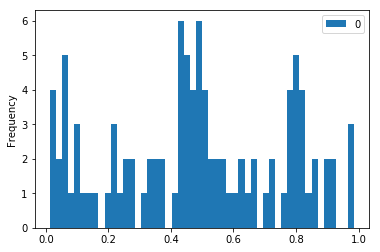# Histograms In Pandas

## Goal¶

This post aims to introduce how to create histogram plot using pandas

## Libraries¶

In :
import pandas as pd
import numpy as np
import matplotlib.pyplot as plt
%matplotlib inline


## Create data¶

In :
df = pd.DataFrame(np.random.random(100))

Out:
0
0 0.984265
1 0.862053
2 0.360947
3 0.146953
4 0.439591

## Create Histogram¶

In :
df.plot(kind='hist', bins=50);